Next: Ecliptic Circle Up: Spherical Astronomy Previous: Celestial Motions

# Celestial Coordinates

Consider Fig. 3. The celestial sphere rotates about the celestial axis,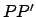, which is the imagined extension of the earth's axis of rotation. This axis intersects the celestial sphere at the north celestial pole,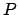, and the south celestial pole,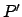. It follows that the two celestial poles are unaffected by diurnal motion, and remain fixed in the sky.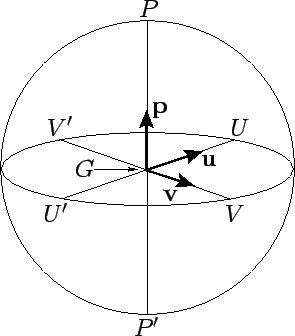The celestial equator,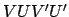, is the intersection of the earth's equatorial plane with the celestial sphere, and is therefore perpendicular to the celestial axis. The so-called vernal equinox,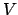, is a particular point on the celestial equator that is used as the origin of celestial longitude. Furthermore, the autumnal equinox,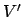, is a point which lies directly opposite the vernal equinox on the celestial equator. Let the line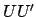lie in the plane of the celestial equator such that it is perpendicular to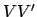, as shown in the figure.

It is helpful to define three, right-handed, mutually perpendicular, unit vectors: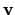,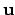, and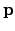. Here,is directed from the earth to the vernal equinox,from the earth to point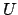, andfrom the earth to the north celestial pole--see Fig. 3.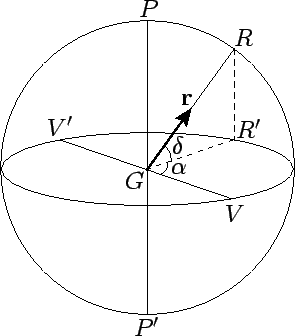Consider a general celestial object,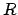--see Fig. 4. The location ofon the celestial sphere is conveniently specified by two angular coordinates,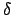and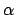. Let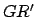be the projection of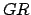onto the equatorial plane. The coordinate, which is known as declination, is the angle subtended betweenand. Objects north of the celestial equator have positive declinations, and vice versa. It follows that objects on the celestial equator have declinations of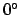, whereas the north and south celestial poles have declinations of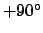and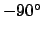, respectively. The coordinate, which is known as right ascension, is the angle subtended between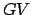and. Right ascension increases from west to east (i.e., in the opposite direction to the celestial sphere's diurnal rotation). Thus, the vernal and autumnal equinoxes have right ascensions ofand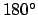, respectively. Note thatlies in the rangeto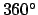. Right ascension is sometimes measured in hours, instead of degrees, with one hour corresponding to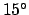(since it takes 24 hours for the celestial sphere to complete one diurnal rotation). In this scheme, the vernal and autumnal equinoxes have right ascensions of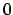hrs. and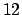hrs., respectively. Moreover,lies in the rangeto 24 hrs. (Incidentally, in this treatise,is measured relative to the mean equinox at date, unless otherwise specified.) Finally, let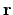be a unit vector which is directed from the earth to--see Fig. 4. It is easily demonstrated that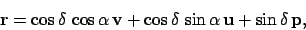(1)

and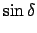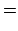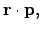(2)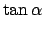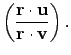(3)Next: Ecliptic Circle Up: Spherical Astronomy Previous: Celestial Motions
Richard Fitzpatrick 2010-07-21